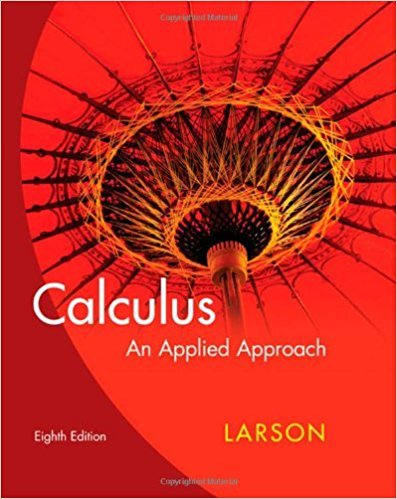×
×

# Solutions for Chapter 10.3: p-Series and the Ratio Test## Full solutions for Calculus: An Applied Approach | 8th Edition

ISBN: 9780618958252Solutions for Chapter 10.3: p-Series and the Ratio Test

Solutions for Chapter 10.3
4 5 0 341 Reviews
30
5
##### ISBN: 9780618958252

This expansive textbook survival guide covers the following chapters and their solutions. Chapter 10.3: p-Series and the Ratio Test includes 66 full step-by-step solutions. This textbook survival guide was created for the textbook: Calculus: An Applied Approach , edition: 8. Since 66 problems in chapter 10.3: p-Series and the Ratio Test have been answered, more than 22692 students have viewed full step-by-step solutions from this chapter. Calculus: An Applied Approach was written by and is associated to the ISBN: 9780618958252.

Key Calculus Terms and definitions covered in this textbook
• Algebraic expression

A combination of variables and constants involving addition, subtraction, multiplication, division, powers, and roots

• Binomial

A polynomial with exactly two terms

• Bounded

A function is bounded if there are numbers b and B such that b ? ƒ(x) ? B for all x in the domain of f.

• Central angle

An angle whose vertex is the center of a circle

• Control

The principle of experimental design that makes it possible to rule out other factors when making inferences about a particular explanatory variable

• Cubic

A degree 3 polynomial function

• Damping factor

The factor Ae-a in an equation such as y = Ae-at cos bt

• Determinant

A number that is associated with a square matrix

• Distance (in a coordinate plane)

The distance d(P, Q) between P(x, y) and Q(x, y) d(P, Q) = 2(x 1 - x 2)2 + (y1 - y2)2

• End behavior

The behavior of a graph of a function as.

• Grapher or graphing utility

Graphing calculator or a computer with graphing software.

See Polynomial function in x

• Linear factorization theorem

A polynomial ƒ(x) of degree n > 0 has the factorization ƒ(x) = a(x1 - z1) 1x - i z 22 Á 1x - z n where the z1 are the zeros of ƒ

• LRAM

A Riemann sum approximation of the area under a curve ƒ(x) from x = a to x = b using x1 as the left-hand endpoint of each subinterval

• Power-reducing identity

A trigonometric identity that reduces the power to which the trigonometric functions are raised.

• Reference triangle

For an angle ? in standard position, a reference triangle is a triangle formed by the terminal side of angle ?, the x-axis, and a perpendicular dropped from a point on the terminal side to the x-axis. The angle in a reference triangle at the origin is the reference angle

• Relation

A set of ordered pairs of real numbers.

• Scatter plot

A plot of all the ordered pairs of a two-variable data set on a coordinate plane.

• Simple harmonic motion

Motion described by d = a sin wt or d = a cos wt

• Weighted mean

A mean calculated in such a way that some elements of the data set have higher weights (that is, are counted more strongly in determining the mean) than others.

×